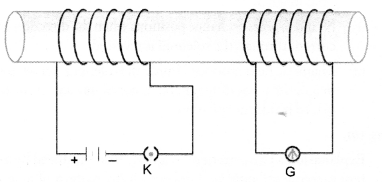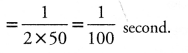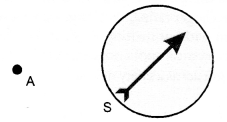## NCERT Exemplar Solutions for Class 10 Science Chapter 13 Magnetic Effects of Electric Current

These Solutions are part of NCERT Exemplar Solutions for Class 10 Science. Here we have given NCERT Exemplar Solutions for Class 10 Science Chapter 13 Magnetic Effects of Electric Current

Question 1.
Choose the incorrect statement from the following regarding magnetic lines of field.
(a) The direction of magnetic field at a point is taken to be the direction in which the north pole of a magnetic compass needle points.
(b) Magnetic field lines are closed curves.
(c) If magnetic field lines are parallel and equidistant, they rep-resent zero field strength.
(d) Relative strength of magnetic field is shown by the degree of closeness of the field lines.
(c).
Explanation : Magnetic field lines which are parallel and equidistant represent uniform magnetic field.

More Resources

Question 2.
If the key in the arrangement shown in figure is taken out (the circuit is made open) and magnetic field lines are drawn over the horizontal plane ABCD, the lines are
(a) concentric circles
(b) elliptical in shape
(c) straight lines parallel to each other
(d) concentric circles near the point O but of elliptical shapes as we go away from it
(c).Explanation : When no current flows through the wire, mag¬netic field lines over the plane ABCD will represent magnetic field of the earth. These field lines are straight lines parallel to each other.

Question 3.
A circular loop placed in a plane perpendicular to the plane of paper carries a current when the key is ON. The current is seen from points A and B (in the plane of the paper and on the axis of the coil) is anticlockwise and clockwise respectively. The magnetic field lines point from B to A. The N-pole of the resultant magnet is on the face close to
(a) A
(b) B
(c) A if current is small, and B if current is large.
(d) B if the current is small, and A if current is large.(a).
Explanation : If current seems to flow in anticlockwise direction in a coil, then that face of the coil acts as N-pole.

Question 4.
In a long straight solenoid, N-and S-poles are created at the two ends of the solenoid. Which of the following statements is incor¬rect ?
(a) The field lines inside the solenoid are in the form of straight lines indicating that magnetic field is same at ail points inside the solenoid.
(b) The pattern of the magnetic field associated with the solenoid is different from the pattern of the magnetic field around a bar magnet.
(c) N-and S-poles exchange positions when the direction of the current through the solenoid is reversed.
(d) Magnetic field produced inside the solenoid can be used to magnetise a bar of magnetic material like soft iron, when placed inside the solenoid.
(b).
Explanation : The pattern of magnetic field produced by a cur¬rent carrying solenoid resembles with the pattern of magnetic field of a bar magnet.

Question 5.
A uniform magnetic field exists in the plane of paper pointing from left to right as shown in figure. In the field, an electron and a proton move as shown. The electron and proton experience(a) forces, both pointing into the plane of paper
(b) forces, both pointing out of the plane of paper
(c) forces, pointing into the plane of paper and out of the plane of paper respectively.
(d) forces, pointing opposite and along the direction of the uniform magnetic field respectively.
(a).
Explanation : Apply Fleming’s left hand rule and considering the flow of current in the direction of the movement of proton and in the direction opposite to the movement of electron.

Question 6.
Commercial electric motors do not use
(a) an electromagnet to rotate the armature
(b) effectively large number of turns of conducting wire in the current carrying coil
(c) a permanet magnet to rotate the armature id a soft iron on which the coil is wound.
(c).
Explanation : In commercial motor, armature rotates in the mag-netic field produced by an electromagnet. The strength of such magnetic field can be increased to the desired value. However, a permanent magnet provides a fixed and weak magnetic field.

Question 7.
Figure shows the arrangment of two coils wound on a non-conducting cylindrical rod. The plugh of the key is then in¬serted and later removed.(a) Deflection in the galvanometer remains zero throughout
(b) There is momentary deflection in the galvanometer that dies out shortly and deflections in both the cases are in the same direction
(c) There is momentary deflection in the galvanometer that dies out shortly and there is no effect when the key is re¬moved
(d) There are momentary deflections in both the cases that die out shortly but the deflections are in apposite directions.
(a).
Explanation : Due to electromagnetic induction, induced cur¬rent flows in the coil with which galvanometer is connected, when the key is inserted (i.e. current grows in the primary coil) and when the key is removed (i.e. current decays in the primary coil).

Question 8.
Choose the incorrect statement
(a) Fleming’s right hand rule is a simple rule to know the direction of induced current.
(b) The right-hand thumb rule is used to find the direction of magnetic fields due to current carrying conductors.
(c) The difference between the direct and alternating currents is that the direct current always flows in one direction, whereas the alternating current reverses its direction periodically.
(d) In India, the AC changes direction after every 1/60 seconds
(d).
Explanation : Frequency of AC in India = 50 Hz
∴ AC completes 1 cycle in 1/50 seconds.
In one cycle, AC changes direction two times. Hence, time after which AC changes directionQuestion 9.
A constant current flows in a horizontal wire in the plane of the paper from east to west as shown in figure. The direction of magnetic field at a point will be north to south.(a) directly below the wire
(b) directly above the wire
(c) at a point located in the plane of the paper, on the north side of the wire
(d) at a point located in the plane of the paper, on the south side of the wire.
(d).
Explanation : Applying right hand thumb rule to know the direction of the magnetic field.

Question 10.
The strength of magnetic field inside a long current carrying straight solenoid is
(a) more at the ends than at the centre
(b) minimum in the middle
(c) same at all points
(d) found to increase from one end to the other.
(c).
Explanation : Magnetic field inside a long current carrying straight solenoid is uniform.

Question 11.
To convert AC generator to DC generator
(a) slip rings and brushes must be used ‘
(b) split ring type commutator must be used
(c) rectangular wire loop is to be used
(d) stronger magnetic field is to be used.
(b).

Question 12.
The most important safety device method used for protecting electrical appliances from short circuiting or overloading is
(a) earthing
(b) use of stabilizers
(c) use of electric meter
(d) use of fuse.
(d).
Explanation : Fuse melts and the circuit breaks as soon as the current in the circuit exceeds safe limit.

Question 13.
A magnetic compass needle is placed in a plane of paper near point A as shown in figure.
In which plane should a straight current carrying conductor be placed so that it passes through A and there is no change in the deflection of the compass ? Under what condition is the deflection maximum and why?The straight current carrying conductor should be placed in the plane of the paper. The deflection of the compass is maxi¬mum when the conductor passes through A and perpendicular to the plane of the paper. The magnetic field due to current carrying conductor is maximum in the plane of the paper.

Question 14.
Under what conditions permanent electromagnet is obtained if a current carrying solenoid is used ? Support your answer with the help of a labelled circuit diagram.
Permanent electromagnet is obtained if

1. direct current flows through the solenoid and
2. steel rod (a magnetic substance) is placed inside the solenoid.

In other words, a steel rod can be made a permanent electro-magnet if it is placed inside a solenoid carrying direct current as shown in figure.Question 15.
AB is a current carrying conductor in the plane of the paper as shown in figure. What are the directions of magnetic fields produced by it at points P and Q ? Given, r1 > r2, where will the strength of the magnetic field be larger ?
(CBSE 2010, 2012)According to Right hand thumb rule, the direction of the mag¬netic field is into the plane of the paper at P and the direction of the magnetic field is out of the plane of the paper at Q.
The strength of the magnetic field due to a current carrying conductor decreases with increase in distance (i.e. B ∝ 1/r ).
Therefore, strength of the magnetic field will be larger at point Q than at point P.

Question 16.
A magnetic compass shows a deflection when placed near a current carrying wire. How will the deflection of the compass get affected if the current in the wire is increased ? Support your answer with a reason. (CBSE 2012)
A magnetic compass is a tiny magnet. It gets deflected when placed in a magnetic field. When the current in the wire increases, then the magnetic field produced by the wire also increases ( B ∝ I). Hence, deflection of the compass increases.

Question 17.
It is established that an electric current through a metallic conductor produces a magnetic field around it. Is there a similar magnetic field produced around a thin beam of moving

1. alpha particles,
(CBSE 2012)

1. A thin beam of moving alpha particles constitute an electric current. Thus, beam of moving alpha particles behaves as a current carrying conductor. Hence, magnetic field is produced around the thin beam of moving alpha particles.
2. Neutrons are neutral particles i.e. they do not have any charge. Therefore, moving neutrons do not constitute an electric cur-rent. Hence, no magnetic field is produced around the thin beam of moving neutrons.

Question 18.
What does the direction of thumb indicate in the right-hand thumb rule. In what way this rule is different from Fleming’s left-hand rule ? (CBSE 2010,2012)
In the right hand rule, the direction of thumb indicates the direction of flow of current in the straight conductor.
Right-hand thumb rule is used to determine the direction of the magnetic field produced by a current carrying straight conductor. However, Flemings left hand rule is used to determine the direction of the force experienced by a current carrying conductor placed in a uniform magnetic field.

Question 19.
Meena draws magnetic field lines of field close to the axis of a current carrying circular loop. As she moves away from the centre of the circular loop, she observes that the lines keep on diverging. How will you explain her observation ? (CBSE 2010)
Magnetic field due to current carrying circular loop decreases as we move away from the centre of the loop. Diverging field lines represent weak magnetic field.

Question 20.
What does the divergence of magnetic field lines near the ends of a current carrying straight solenoid indicate ?
Divergence of magnetic field lines means the magnetic field lines are far apart. Such field lines indicate weak magnetic field. There¬fore, divergence of magnetic field lines near the ends of the current carrying solenoid indicates that the magnetic field near the ends of the current carrying solenoid is weak and non-uniform.

Question 21.
Name four appliances wherein an electric motor, a rotating device that converts electrical energy to mechanical energy is used as an important component. In what respect motors are different from generators ?
Appliances wherein an electric motor is used are

1. electric fan,
2. electric mixer,
3. washing machine and
4. a toy car.

Motor converts electrical energy into mechanical energy. How-ever, generator converts mechanical energy into electrical energy.

Question 22.
What is the role of two conducting stationary brushes in a simple electric motor ?
These brushes act as the contacts between the commutator and the terminals of the battery.

Question 23.
What is the difference between direct current and an alternating current ? How many times does AC used in India change direction in one second ?
Difference between Direct current and an Alternating current:

 Direct current Alternating current 1. The magnitude of direct current is constant and flows in one direction only. 2. Direct current cannot be used for large scale supply of electricity for household purpose. 3. The frequency of direct current is zero. 1. The magnitude of AC changes with time and direction of alternating current reverses periodically. 2. Alternating current is used to run electrical appliances like electric bulb, electric heater, electric iron, refrigerator etc. 3. Frequency of alternating current in India is 50 Hz.

Since frequency is 50 Hz, so a.c. completes 50 cycles in 1 second. Therefore, it reverses its direction 100 times in one second.

Question 24.
What is the role of fuse, used in series with any electrical appli-ances ? Why should a fuse with defined rating not be replaced by one with a larger rating ?